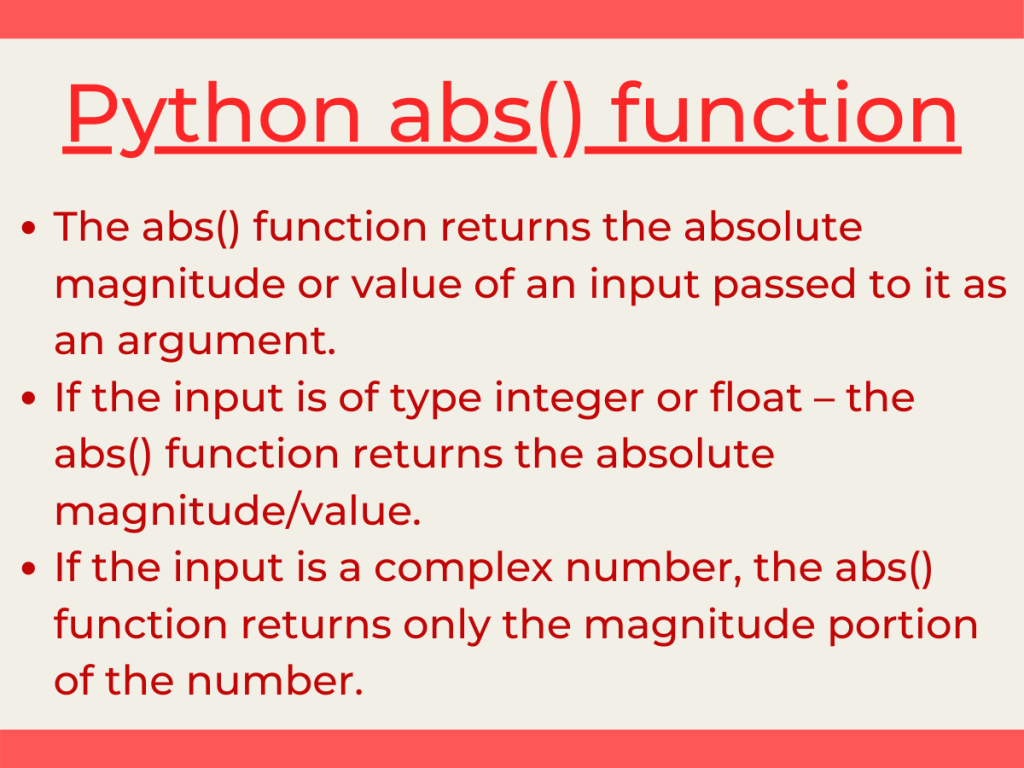# Python abs() functionHey, folks! In this article, we will be unveiling Python abs() function with different modules such as NumPy and Pandas.

## Getting started with Python abs() function

Python has a huge number of in-built functions to deal with mathematical and statistical operations. One such function is the Python abs() function.

The `abs() function` returns the absolute magnitude or value of input passed to it as an argument. It returns the actual value of input without taking the sign into consideration.

The abs() function accepts only a single arguement that has to be a number and it returns the absolute magnitude of the number.

• If the input is of type `integer` or `float `– the abs() function returns the absolute magnitude/value
• If the input is a `complex number`, the abs() function returns only the magnitude portion of the number

Syntax:

```abs(number)
```
• The number can be of integer type, floating point type or a complex number.

Example:

```num = -25.78
print("Absolute value:",abs(num))
```

Output:

```Absolute value: 25.78
```

## Pandas DataFrame.abs() function

Python Pandas module has in-built `DataFrame.abs() function` to calculate the absolute value of all the data values present in a particular data variable/column of a data frame of a data set.

Syntax:

```DataFrame['column_name'].abs()
```

Input Dataset:

Example:

```import pandas as pd
import numpy as np
data['cons.conf.idx'].abs()
```

In the above snippet of code, we have used `pandas.read_csv() function` to import and load the dataset into the environment. The `DataFrame.head(n) function` actually represents the first ‘n’ values of the dataset.

Further, we have found out the absolute values of the column ‘cons.conf.idx‘ using the abs() function.

Output:

```0       42.0
1       42.7
2       36.4
3       42.7
4       46.2
...
7409    36.4
7410    42.7
7411    46.2
7412    42.0
7413    36.4
Name: cons.conf.idx, Length: 7414, dtype: float64
```

## Python numpy.absolute() function

Python NumPy module has `numpy.absolute() function` to fetch the absolute values of array elements passed to it.

Syntax:

```numpy.absolute(array)
```

Example:

```import numpy as np
arr = [10, -20, 30, -40]
abs_res = np.absolute(arr)
print("The absolute values of the array elements:",abs_res)
```

Output:

```The absolute values of the array elements: [10 20 30 40]
```

## Conclusion

Thus, in this article, we have understood the implementation of Python abs() function with NumPy and Pandas module.